# PyTorch中交叉熵的计算-CrossEntropyLoss 介绍2020年4月9日07:10:44

6 3303字阅读11分0秒

## CrossEntropyLoss 的理解

### LogSoftmax 的介绍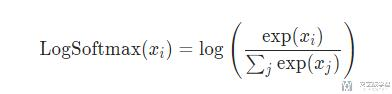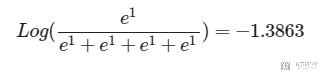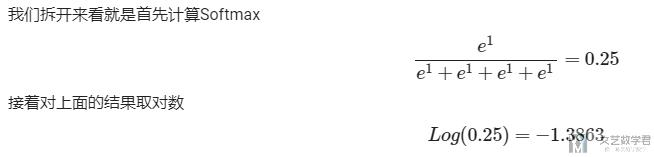1. ll = nn.LogSoftmax()
2. input = torch.tensor([1.0,1.0,1.0,1.0])
3. output = ll(input)
4. print(output)
5. >> tensor([-1.3863, -1.3863, -1.3863, -1.3863])

### NLLLoss的介绍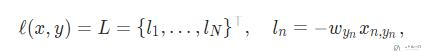1. loss = nn.NLLLoss()
2. input = torch.tensor([[0.2, 0.3, 0.5]])
3. target = torch.tensor().long()
4. output = loss(input, target)
5. print(output)
6. >> tensor(-0.5000)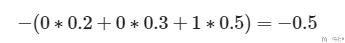## Pytorch使用LogSoftmax的原因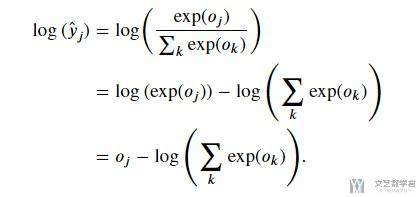## CrossEntropyLoss的介绍

### 交叉熵计算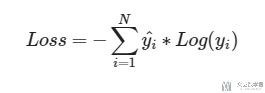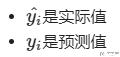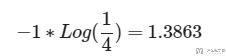### 关于PyTorch中的CrossEntropyLoss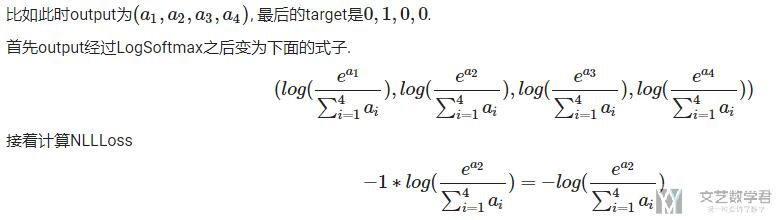1. loss = nn.NLLLoss()
2. Y = torch.tensor([2, 1, 0])
3. data = torch.tensor(
4.     [[0.3, 0.3, 0.4],
5.      [0.3, 0.4, 0.3],
6.      [0.1, 0.2, 0.7]])
7. loss(data, Y)
8. # >> tensor(-0.3000)

### CrossEntropyLoss实验验证

1. loss = nn.CrossEntropyLoss()
2. input = torch.tensor([[1, 1, 1.0, 1]])
3. target = torch.tensor().long()
4. output = loss(input, target)
5. print(output)
6. >> tensor(1.3863)

• 当预测的概率为(1,0,0), 实际值(target)是(1,0,0)的时候, 交叉熵是接近0的.
• 当预测的概率为(0,0,1), 实际值(target)是(1,0,0)的时候, 交叉熵是接近正无穷的.
1. # 预测和实际很接近
2. loss = nn.CrossEntropyLoss()
3. input = torch.tensor([[0, 0, 100.0]])
4. target = torch.tensor().long()
5. output = loss(input, target)
6. print(output)
7. >> tensor(0.)

1. # 预测和实际差很远
2. loss = nn.CrossEntropyLoss()
3. input = torch.tensor([[100.0, 0, 0]])
4. target = torch.tensor().long()
5. output = loss(input, target)
6. print(output)
7. >> tensor(100.)

### 一些可能出现的问题

1. CrossEntropy in Pytorch getting Target 1 out of bounds

nn.CrossEntropyLoss is used for a multi-class classification, while your model outputs the logits for a single class. (出现报错的原因, 在一个二分类的问题中) If you are dealing with a binary classification, you could use nn.BCEWithLogitsLoss, or output two logits and keep nn.CrossEntropyLoss. (使用CrossEntropyLoss的时候, 网络的output就是要有2, linear的output的维度是2)
• 微信公众号
• 关注微信公众号
•• QQ群
• 我们的QQ群号
•• 本文由 发表于 2020年4月9日07:10:44
• 转载请务必保留本文链接：https://mathpretty.com/12068.html